### CE2351 Structural Analysis–II Two Marks with Answers for May June 2013 Exam

Anna University

DEPARTMENT OF CIVIL ENGINEERING

CE 1352 – STRUCTURAL ANALYSIS - II

(Two Marks)

UNIT - I

FLEXIBILITY METHOD FOR INDETERMINATE FRAMES

1. What is meant by indeterminate structures?

Structures that do not satisfy the conditions of equilibrium are called indeterminate structure. These structures cannot be solved by ordinary analysis techniques.

2. What are the conditions of equilibrium?

The three conditions of equilibrium are the sum of horizontal forces, vertical forces and moments at any joint should be equal to zero.

3. Differentiate between determinate and indeterminate structures.Determinate structures can be solving using conditions of equilibrium alone ( H = 0; V = 0; M = 0). No other conditions are required.

Indeterminate structures cannot be solved using conditions of equilibrium because ( H ≠ 0; V ≠0; M ≠ 0). Additional conditions are required for solving such structures.

4. Define degree of indeterminacy.

The excess number of reactions that make a structure indeterminate is called degree of

Indeterminacy. Indeterminacy is also called degree of redundancy.

Indeterminacy consists of internal and external indeterminacies.

It is denoted by the symbol ‘i’

Degree of redundancy (i) = I.I. + E.I.

Where I.I. = internal indeterminacy and E.I. = external indeterminacy.

5. Define internal and external indeterminacies.

Internal indeterminacy (I.I.) is the excess no of internal forces present in a member that make a structure indeterminate.

External indeterminacy (E.I.) is excess no of external reactions in the member that make the structure indeterminate.

Indeterminacy = I.I. + E.I.

E.I. = r – e; where r = no of support reactions and e = equilibrium conditions

I.I. = i – EI

e = 3 (plane frames) and e = 6 (space frames)

6. Write the formulae for degree of indeterminacy for:

(a) Two dimensional pinjointed truss (2D Truss)

i = (m+r) – 2j where m = no of members

r = no of reactions; j = no of joints

(b) Two dimensional rigid frames/plane rigid frames (2D Frames)

i = (3m+r) – 3j where m = no of members

r = no of reactions; j = no of joints

(c) Three dimensional space truss (3D Truss)

i = (m+r) – 3j where m = no of members

r = no of reactions; j = no of joints

(d) Three dimensional space frames (3D Frame)

i = (6m+r) – 6j where m = no of members

r = no of reactions; j = no of joints

7. Determine the degree of indeterminacy for the following 2D truss.

i = (m+r) - 2j

Where, m = 19; r = 4; j = 10; e = 3 i = (19+4) – 2 x 10 = 3

External indeterminacy E.I. = r – e = 4 – 3 = 1

Internal indeterminacy I.I. = i – E.I. = 3 -1 = 2

8. Determine the total, internal and external degree of indeterminacy for the plane rigid frame below.

i = (3m + r) – 3j

Where, m = 7; r = 4; j = 6; e = 3 i = (3 x 7 + 4) – (3 x 6) = 7

External indeterminacy E.I. = r – e = 4 – 3 = 1

Internal indeterminacy I.I. = i – EI = 7 -1 = 6

9. Determine i, EI, II for the given plane truss.

i = (m + r) – 2j

Where, m = 3; r = 4; j = 3; e = 3 i = (3 + 4) – (2 x 3) = 1

External indeterminacy EI = r – e = 4 – 3 = 1

Internal indeterminacy II = i – EI = 1 -1 = 0

10. Find the indeterminacy for the beams given below.

For beams degree of indeterminacy is given by i = r – e

i = r – e where, r = no of reactions, e = no of equilibrium conditions r = 4 and e = 3

i = 4 – 3 = 1

i = r – e where, r = no of reactions, e = no of equilibrium conditions r = 5 and e = 3

i = 5 – 3 = 2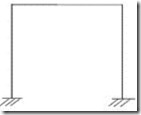11. Find the indeterminacy for the given rigid plane frame.

i = (3m + r) – 3j

Where, m = 3; r = 4; j = 4

i = (3 x 3 + 4) – (3 x 4) = 1

External indeterminacy EI = r – e = 4 – 3 = 1

Internal indeterminacy II = i – EI = 1 - 1 = 0

12. Find the indeterminacy of the space rigid frame.

i = (6m + r) – 6j

Where, m = 8; r = 24 (i. e. 6 per support x 4)

j = 8; e = 6

i = (6 x 8 + 24) – (6 x 8) = 24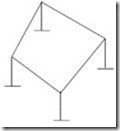External indeterminacy EI = r – e = 24 – 6 = 18

Internal indeterminacy II = i – EI = 24 -18 = 6

13. Find the indeterminacy for the given space truss.

i = m + r – 3j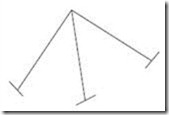Where m = 3

r = 18 (i. e. 6 reactions per support x 3)

j = 4

i = (3 + 18) – (3 x 4) = 9

External indeterminacy EI = r – e = 18 – 6 = 12

Internal indeterminacy II = i – EI = 9 -12 = –3

14. What are the different methods of analysis of indeterminate structures?

The various methods adopted for the analysis of indeterminate structures include:

v Flexibility matrix method

v Stiffness matrix method

v Finite Element method

15. Briefly mention the two types of matrix methods of analysis of indeterminate structures.

The two matrix methods of analysis of indeterminate structures are: (i) Flexibility matrix method:

This method is also called the force method in which the forces in the structure are treated as unknowns. The no of equations involved is equal to the degree of static indeterminacy of the structure.

(ii) Stiffness matrix method:

This is also called the displacement method in which the displacements that occur in the structure are treated as unknowns. The no of displacements involved is equal to the no of degrees of freedom of the structure.

16. Define a primary structure.

A structure formed by the removing the excess or redundant restraints from an indeterminate structure making it statically determinate is called primary structure. This is required for solving indeterminate structures by flexibility matrix method.

17. Define kinematic indeterminacy (Dk) or Degree of Freedom (DOF)

Degree of freedom is defined as the least no of independent displacements required to define the deformed shape of a structure.

There are two types of DOF: (a) Nodal type DOF and (b) Joint type DOF.

18. Briefly explain the two types of DOF.

(a) Nodal type DOF – This includes the DOF at the point of application of concentrated load or moment, at a section where moment of inertia changes, hinge support, roller support and junction of two or more members.

(b) Joint type DOF – This includes the DOF at the point where moment of inertia changes, hinge and roller support, and junction of two or more members.

19. For the truss shown below, what is the DOF?

Pin-jointed plane frame/truss

DOF / Dk = 2j – r

Where, r = no of reactions; j = no of joints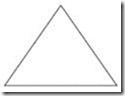20. Define compatibility in force method of analysis .

Compatibility is defined as the continuity condition on the displacements of the structure after external loads are applied to the structure.

21. Define the Force Transformation Matrix.

The connectivity matrix which relates the internal forces Q and the external forces R is known as the force transformation matrix. Writing it in a matrix form,

{Q} = [b] {R}

Where, Q = member force matrix/vector; b = force transformation matrix

R = external force/load matrix/ vector

22. What are the requirements to be satisfied while analyzing a structure?

The three conditions to be satisfied are: (i) Equilibrium condition

(ii) Compatibility condition

(iii) Force displacement condition

23. Define flexibility influence coefficient (fij)

Flexibility influence coefficient (fij) is defined as the displacement at joint ‘i’ due to a unit load at

joint ‘j’, while a l other joints are not load.

24. Write the element flexibility matrix (f) for a truss member & for a beam element.

The element flexibility matrix (f) for a truss member is given by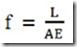The element flexibility matrix (f) for a beam element is given by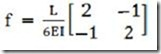U

NIT - II

MATRIX STIFFNESS METHOD

1. What are the basic unknowns in stiffness matrix method?

In the stiffness matrix method nodal displacements are treated as the basic unknowns for the solution of indeterminate structures.

2. Define stiffness coefficient kij.

Stiffness coefficient ‘kij’ is defined as the force developed at joint ‘i’ due to unit displacement at

joint ‘j’ while a l other joints are fixed.

3. What is the basic aim of the stiffness method?

The aim of the stiffness method is to evaluate the values of generalized coordinates ‘r’ knowing

the structure stiffness matrix ‘k’ and nodal loads ‘R’ through the structure equilibrium equation.

{R} = [K] {r}

4. What is the displacement transformation matrix?

The connectivity matrix which relates the internal displacement ‘q’ and the external displacement

‘r’ is known as the displacement transformation matrix ‘a’.

{q} = [a] {r}

5. How are the basic equations of stiffness matrix obtained?

The basic equations of stiffness matrix are obtained as: Equilibrium forces

Compatibility of displacements

Force displacement relationships

6. What is the equilibrium condition used in the stiffness method?

The external loads and the internal member forces must be in equilibrium at the nodal points.

7. What is meant by generalized coordinates?

For specifying a configuration of a system, a certain minimum no of independent coordinates are necessary. The least no of independent coordinates that are needed to specify the configuration is known as generalized coordinates.

8. What is the compatibility condition used in the flexibility method?

The deformed elements fit together at nodal points.

9. Write about the force displacement relationship.

The relationship of each element must satisfy the stress-strain relationship of the element material.

10. Write the element stiffness for a truss element.

The element stiffness matrix for a truss element is given by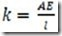11. Write the element stiffness matrix for a beam ele ment.

The element stiffness matrix for a beam element is given by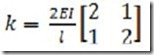12. Compare flexibility method and stiffness method.

Flex ibility matrix method:

The redundant forces are treated as basic unknowns.

The number of equations involved is equal to the degree of static indeterminacy of the structure.

The method is the generalization of consistent deformation method. Different procedures are used for determinate and indeterminate structures

Stiffness matrix method:

The joint displacements are treated as basic unknowns

The number of displacements involved is equal to the no of degrees of freedom of the

structure

The method is the generalization of the slope deflection method.

The same procedure is used for both determinate and indeterminate structures.

13. Is it possible to develop the flexibility matrix for an unstable structure?

In order to develop the flexibility matrix for a structure, it has to be stable and determinate.

14. What is the relation between flexibility and stiffness matrix?

The element stiffness matrix ‘k’ is the inverse of the element flexibility matrix ‘f’ and is given by f = 1/k or k = 1/f.

15. What are the types of structures that can be solved using stiffness matrix method?

Structures such as simply supported, fixed beams and portal frames can be solved using stiffness matrix method.

16. Give the formula for the size of the Global stiffness matrix.

The size of the global stiffness matrix (GSM) = No: of nodes x Degrees of freedom per node.

17. List the properties of the stiffness matrix

The properties of the stiffness matrix are: It is a symmetric matrix

The sum of elements in any column must be equal to zero.

It is an unstable element therefore the determinant is equal to zero.

18. Why is the stiffness matrix method also called equilibrium method or displacement method?

Stiffness method is based on the superposition of displacements and hence is also known as the displacement method. And since it leads to the equilibrium equations the method is also known as equilibrium method.

19. If the flexibility matrix is given as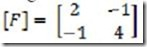Write the corresponding stiffness matrix.

Stiffness matrix = 1 / (Flexibility matrix)

i.e. [K] = [F]-1

20. Write then stiffness matrix for a 2 D beam element.

The stiffness matrix for a 2 D beam element is given by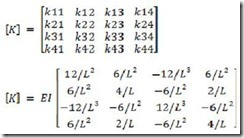UNIT III

FINITE ELEMENT METHOD

1. What is meant by Finite element method?

Finite element method (FEM) is a numerical technique for solving boundary value problems in which a large domain is divided into smaller pieces or elements. The solution is determined by assuming certain polynomials. The small pieces are called finite element and the polynomials are called shape functions.

2. List out the advantages of FEM.

Ø Since the properties of each element are evaluated separately different material properties can be incorporated for each element.

Ø There is no restriction in the shape of the medium.

Ø Any type of boundary condition can be adopted.

3. List out the disadvantages of FEM.

Ø The computational cost is high.

Ø The solution is approximate and several checks are required.

4. Mention the various coordinates in FEM.

Ø Local or element coordinates

Ø Natural coordinates

Ø Simple natural coordinates

Ø Area coordinates or Triangular coordinates

Ø Generalized coordinates

5. What are the basic steps in FEM?

Ø Discretization of the structure

Ø Selection of suitable displacement function

Ø Finding the element properties

Ø Assembling the element properties

Ø Applying the boundary conditions

Ø Solving the system of equations

Ø Computing additional results

6. What is meant by discretization?

Discretization is the process of subdividing the given body into a number of elements which results in a system of equivalent finite elements.

7. What are the factors governing the selection of finite elements?

Ø The geometry of the body

Ø The number of independent space coordinates

8. Define displacement function.

Displacement function is defined as simple functions which are assumed to approximate the displacements for each element. They may assume in the form of polynomials, or trigonometrical functions.

9. Briefly explain a few terminology used in FEM.

The various terms used in FEM are explained below.

Finite element: Small elements used for subdividing the given domain to be analysed are called finite elements. These elements may be 1D, 2D or 3D elements depending on the type of structure.

Nodes and nodal points: The intersection of the different sides of elements are called nodes. Nodes are of two types – external nodes and internal nodes.

External nodes – The nodal point connecting adjacent elements.

Internal nodes – The extra nodes used to increase the accuracy of solution.

Nodal lines: The interface between elements are called nodal lines.

Continuum: The domain in which matter exists at every point is called a continuum. It can be assumed as having infinite number of connected particles.

Primary unk nowns: The main unknowns involved in the formulation of the element properties are known as primary unknowns.

Secondary unk nowns: These unknowns are derived from primary unknowns are known as secondary unknowns. In displacement formulations, displacements are treated as primary unknowns and stress, strain, moments and shear force are treated as secondary unknowns.

10. What are different types of elements used in FEM?

The various elements used in FEM are classified as: One dimensional elements (1D elements) Two dimensional elements (2D elements) Three dimensional elements (3D elements)

11. What are 1-D elements? Give examples.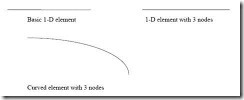Elements having a minimum of two nodes are called 1D element. Beams are usually approximated with 1D element. These may be straight or curved. There can be additional nodes within the element.

12. What are 2-D elements? Give examples.

A plane wall, plate, diaphragm, slab, shell etc. can be approximated as an assemblage of 2-D

elements. Most commonly used elements are triangular, rectangular and quadrilateral elements.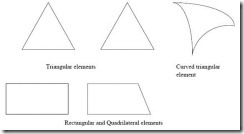13. What are 3-D elements? Give examples.

3-D elements are used for modeling solid bodies and the various 3-D elements are tetrahedron, hexahedron, and curved rectangular solid.

14. Define Shape function.

Shape function is also called an approximate function or an interpolation function whose value is equal to unity at the node considered and zeros at all other nodes. Shape function is represented by Ni where i = node no.

15. What are the properties of shape functions?

The properties of shape functions are:

Ø The no of shape functions will be equal to the no of nodes present in the element.

Ø Shape function will have a unit value at the node considered and zero value at other nodes.

Ø The sum of all the shape function is equal to 1.

16. Define aspect ratio.

Element aspect ratio is defined as the ratio of the largest dimension of the element to its smallest dimension.

17. What are possible locations for nodes?

The possible locations for nodes are:

Ø Point of application of concentrated load.

Ø Location where there is a change in intensity of loads

Ø Locations where there are discontinuities in the geometry of the structure

Ø Interfaces between materials of different properties.

18. What are the characteristics of displacement functions?

Displacement functions should have the following characteristics:

Ø The displacement field should be continuous.

Ø The displacement function should be compatible between adjacent elements

Ø The displacement field must represent constant strain states of elements

Ø The displacement function must represent rigid body displacements of an element.

19. What is meant by plane strain condition?

Plane strain is a state of strain in which normal strain and shear strain directed perpendicular to the plane of body is assumed to be zero.

U

NIT – IV

PLASTIC ANALYSIS OF STRUCTURES

1. What is a plastic hinge?

When a section attains full plastic moment Mp, it acts as hinge which is called a plastic hinge. It is defined as the yielded zone due to bending at which large rotations can occur with a constant value of plastic moment Mp.

2. What is a mechanism?

When a n-degree indeterminate structure develops n plastic hinges, it becomes determinate and the formation of an additional hinge will reduce the structure to a mechanism. Once a structure becomes a mechanism, it will collapse.

3. What is difference between plastic hinge and mechanical hinge?

Plastic hinges modify the behavior of structures in the same way as mechanical hinges. The only difference is that plastic hinges permit rotation with a constant resisting moment equal to the plastic moment Mp. At mechanical hinges, the resisting moment is equal to zero.

4. Define collapse load.

The load that causes the (n + 1) the hinge to form a mechanism is called collapse load where n is the degree of statically indeterminacy. Once the structure becomes a mechanism

5. List out the assumptions made for plastic analysis.

The assumptions for plastic analysis are:

Ø Plane transverse sections remain plane and normal to the longitudinal axis before and after bending.

Ø Effect of shear is neglected.

Ø The material is homogeneous and isotropic both in the elastic and plastic state.

Ø Modulus of elasticity has the same value both in tension and compression.

Ø There is no resultant axial force in the beam.

Ø The cross-section of the beam is symmetrical about an axis through its centroid and parallel to the plane of bending.

6. Define shape factor.

Shape factor (S) is defined as the ratio of plastic moment of the section to the yield moment of the section.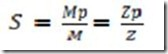Where Mp = Plastic moment

M = Yield moment

Zp = Plastic section modulus

Z = Elastic section modulus

7. List out the shape factors for the following sections.

(a) Rectangular section, S = 1.5

(b) Triangular section, S = 2.346 (c) Circular section, S = 1.697 (d) Diamond section, S = 2

8. Mention the section having maximum shape factor.

The section having maximum shape factor is a triangular section, S = 2.345.

9. Define load factor.

Load factor is defined as the ratio of collapse load to working load and is given by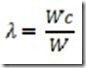10. State upper bound theory.

Upper bound theory states that of all the assumed mechanisms the exact collapse mechanism is that which requires a minimum load.

11. State lower bound theory.

Lower bound theory states that the collapse load is determined by assuming suitable moment distribution diagram. The moment distribution diagram is drawn in such a way that the conditions of equilibrium are satisfied.

12. What are the different types of mechanisms?

The different types of mechanisms are:

Ø Beam mechanism

Ø Column mechanism

Ø Panel or sway mechanism

Ø Cable mechanism

Ø Combined or composite mechanism

13. Mention the types of frames.

Frames are broadly of two types: (a) Symmetric frames

(b) Un-symmetric frames

14. What are symmetric frames and how they analyzed?

Symmetric frames are frames having the same support conditions, lengths and loading conditions on the columns and beams of the frame. Symmetric frames can be analyzed by:

(a) Beam mechanism

(b) Column mechanism

15. What are unsymmetrical frames and how are they analyzed?

Un-symmetric frames have different support conditions, lengths and loading conditions on its columns and beams. These frames can be analyzed by:

(a) Beam mechanism

(b) Column mechanism

(c) Panel or sway mechanism

(d) Combined mechanism

16. Define plastic modulus of a section Zp.

The plastic modulus of a section is the first moment of the area above and below the equal area axis. It is the resisting modulus of a fully plasticized section.

Zp = A/2 (y1 + y2)

17. How is the shape factor of a hollow circular section related to the shape factor of a ordinary circular section?

The shape factor of a hollow circular section = A factor K x shape factor of ordinary circular

section.

SF of hollow circular section = SF of circular section x {(1 – c3)/ (1 – c4)}

18. Give the governing equation for bending.

The governing equation for bending is given by

M/I = /y

Where M = Bending moment

I = Moment of inertia

y = c. g. distance

19. Give the theorems for determining the collapse load.

The two theorems for the determination of collapse load are: (a) Static Method [Lower bound Theorem]

(b) Kinematic Method [Upper bound Theorem]

U

NIT-V

CABLE AND SPACE STRUCTURES

1. What are cable structures?

Long span structures subjected to tension and uses suspension cables for supports. Examples of cable structures are suspension bridges, cable stayed roof.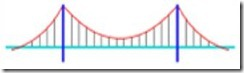Suspension bridge – cable structure

2. What is the true shape of cable structures?

Cable structures especially the cable of a suspension bridge is in the form of a catenary. Catenary is the shape assumed by a string / cable freely suspended between two points.

3. What is the nature of force in the cables?

Cables of cable structures have only tension and no compression or bending.

4. What is a catenary?

Catenary is the shape taken up by a cable or rope freely suspended between two supports and under its own self weight.

5. Mention the different types of cable structures.

Cable structures are mainly of two types: (a) Cable over a guide pulley

(b) Cable over a saddle

6. Briefly explain cable over a guide pulley.

Cable over a guide pulley has the following properties:

Ø Tension in the suspension cable = Tension in the anchor cable

Ø The supporting tower will be subjected to vertical pressure and bending due to net horizontal cable tension.

7. Briefly explain cable over saddle.

Cable over saddle has the following properties:

Ø Horizontal component of tension in the suspension cable = Horizontal component of tension in the anchor cable

Ø The supporting tower will be subjected to only vertical pressure due to cable tension.

8. What are the main functions of stiffening girders in suspension bridges?

Stiffening girders have the following functions.

Ø They help in keeping the cables in shape

Ø They resist part of shear force and bending moment due to live loads.

9. What is the degree of indeterminacy of a suspension bridge with two hinged stiffening girder?

The two hinged stiffening girder has one degree of indeterminacy.

10. Differentiate between plane truss and space truss.

Plane truss:

Ø All members lie in one plane

Ø All joints are assumed to be hinged.

Space truss:

Ø This is a three dimensional truss

Ø All joints are assumed to be ball and socketed.

11. Define tension coefficient of a truss member.

The tension coefficient for a member of a truss is defined as the pull or tension in the member divided by its length, i. e. the force in the member per unit length.

12. Give some examples of beams curved in plan.

Curved beams are found in the following structures.

Ø Beams in a bridge negotiating a curve

Ø Ring beams supporting a water tank

Ø Beams supporting corner lintels

Ø Beams in ramps

13. What are the forces developed in beams curved in plan?

Beams curved in plan will have the following forces developed in them:

Ø Shear forces

Ø Torsional moments

14. What are the significant features of circular beams on equally spaced supports?

Ø Slope on either side of any support will be zero.

Ø Torsional moment on every support will be zero

15. Give the expression for calculating equivalent UDL on a girder.

Equivalent UDL on a girder is given by: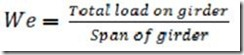16. Give the range of central dip of a cable.

The central dip of a cable ranges from 1/10 to 1/12 of the span.

17. Give the expression for determining the tension T in the cable.

The tension developed in the cable is given byWhere, H = horizontal component and V = vertical component.

18. Give the types of significant cable structures

Linear structures:

Ø Suspension bridges

Ø Cable-stayed beams or trusses

Ø Cable trusses

Ø Straight tensioned cables

Three-dimensional structures:

Ø 3D cable trusses

Ø Tensegrity structures

Ø Tensairity structures

19. What are cables made of?

Cables can be of mild steel, high strength steel, stainless steel, or polyester fibres. Structural cables are made of a series of small strands twisted or bound together to form a much larger cable. Steel cables are either spiral strand, where circular rods are twisted together or locked coil strand, where individual interlocking steel strands form the cable (often with a spiral strand core).

Spiral strand is slightly weaker than locked coil strand. Steel spiral strand cables have a Young's modulus, E of 150 ± 10 kN/mm² and come in sizes from 3 to 90 mm diameter. Spiral strand suffers from construction stretch, where the strands compact when the cable is loaded.

#### 1 comment:

1.Need eyeglasses? With so many options available today, choosing the Best Lenses and Frames for your needs can be overwhelming. So, if you're looking for Best Prescription Glasses or just a Fashion Frame Glasses to boost up your style, ,Check out the Largest Selection of Affordable Glasses Online including
Best Prescription Glasses and Sunglasses for men, women, and kids.